Скачать презентацию Hierarchical Modeling and the Economics of Risk in

fbacdfc59e3f3b8c7342f4d429f4d24f.ppt

• Количество слайдов: 52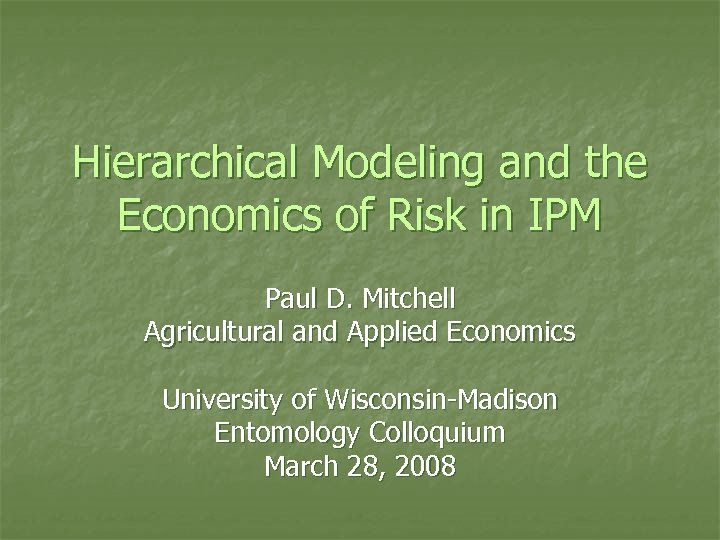Hierarchical Modeling and the Economics of Risk in IPM Paul D. Mitchell Agricultural and Applied Economics University of Wisconsin-Madison Entomology Colloquium March 28, 2008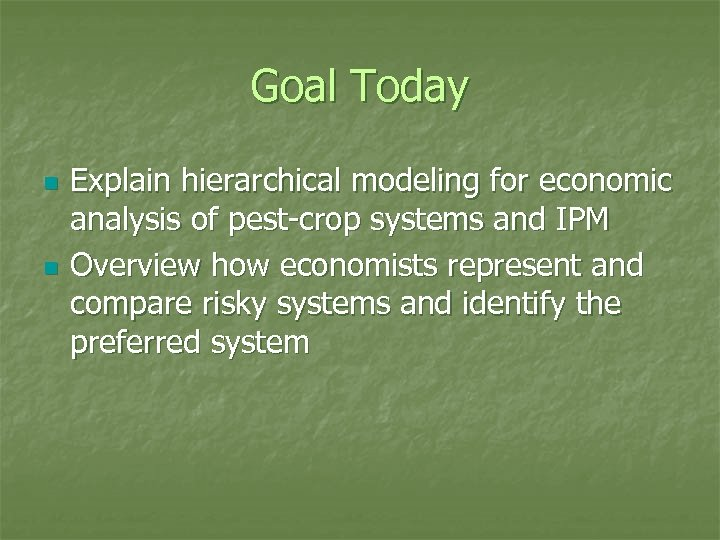Goal Today n n Explain hierarchical modeling for economic analysis of pest-crop systems and IPM Overview how economists represent and compare risky systems and identify the preferred system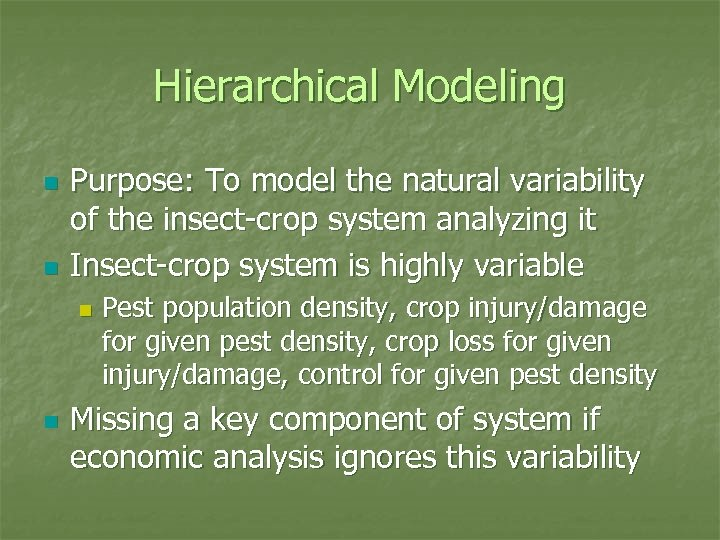Hierarchical Modeling n n Purpose: To model the natural variability of the insect-crop system analyzing it Insect-crop system is highly variable n n Pest population density, crop injury/damage for given pest density, crop loss for given injury/damage, control for given pest density Missing a key component of system if economic analysis ignores this variability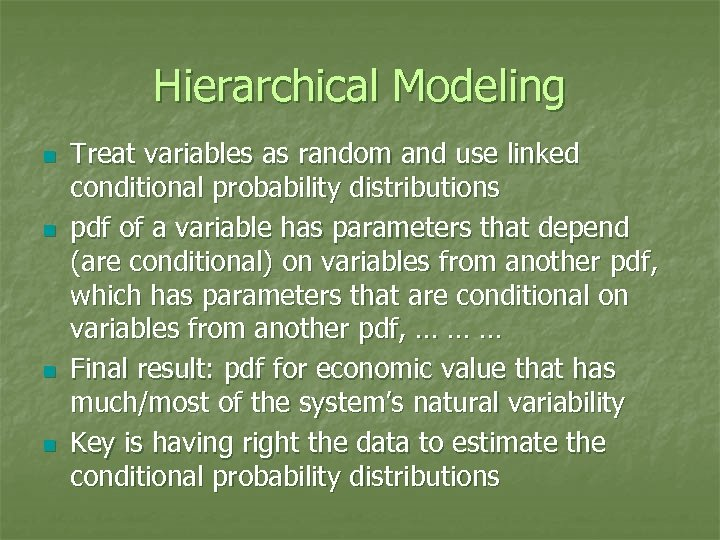Hierarchical Modeling n n Treat variables as random and use linked conditional probability distributions pdf of a variable has parameters that depend (are conditional) on variables from another pdf, which has parameters that are conditional on variables from another pdf, … … … Final result: pdf for economic value that has much/most of the system’s natural variability Key is having right the data to estimate the conditional probability distributions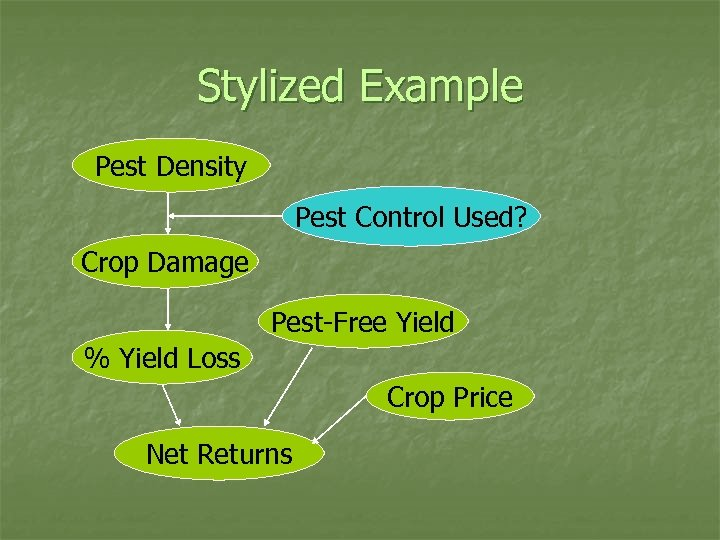Stylized Example Pest Density Pest Control Used? Crop Damage Pest-Free Yield % Yield Loss Crop Price Net Returns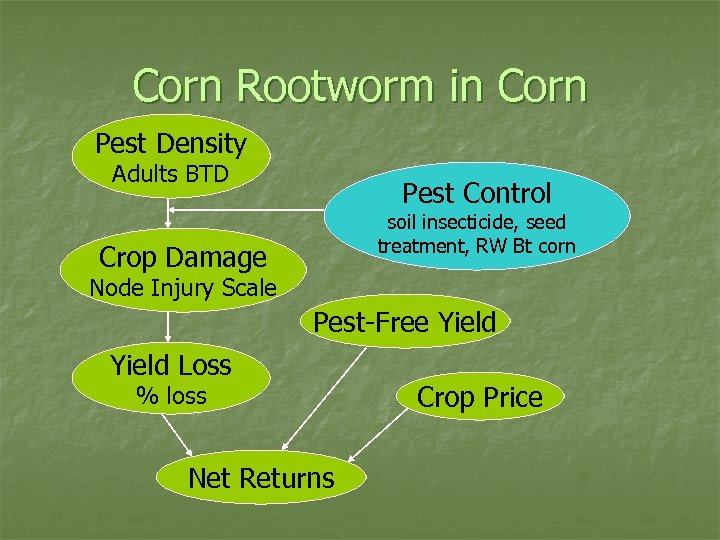Corn Rootworm in Corn Pest Density Adults BTD Pest Control soil insecticide, seed treatment, RW Bt corn Crop Damage Node Injury Scale Pest-Free Yield Loss % loss Net Returns Crop Price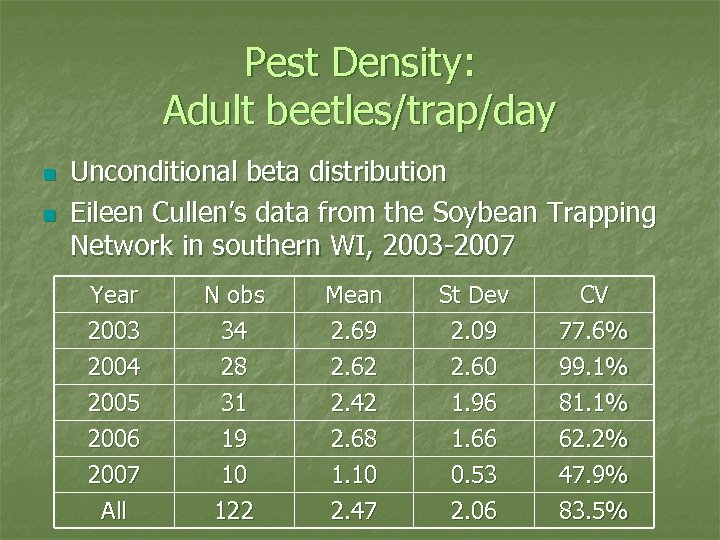Pest Density: Adult beetles/trap/day n n Unconditional beta distribution Eileen Cullen’s data from the Soybean Trapping Network in southern WI, 2003 -2007 Year 2003 2004 2005 Mean 2. 69 2. 62 2. 42 St Dev 2. 09 2. 60 1. 96 CV 77. 6% 99. 1% 81. 1% 2006 2007 N obs 34 28 31 19 10 2. 68 1. 10 1. 66 0. 53 62. 2% 47. 9% All 122 2. 47 2. 06 83. 5%Pest Density: Adult beetles/trap/day Parm Estimate Error t-stat P-value mean 2. 48 0. 18 13. 64 0. 000 st dev 2. 00 0. 15 13. 67 0. 000 max 12. 64 4. 76 2. 66 0. 008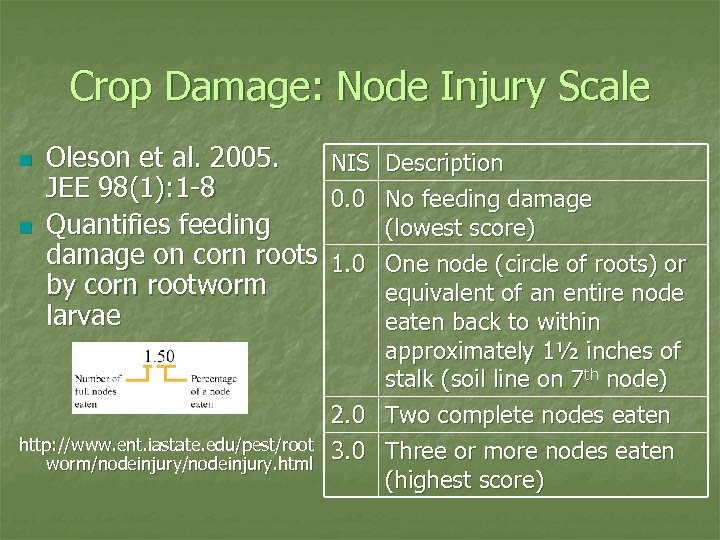Crop Damage: Node Injury Scale n n Oleson et al. 2005. JEE 98(1): 1 -8 Quantifies feeding damage on corn roots by corn rootworm larvae http: //www. ent. iastate. edu/pest/root worm/nodeinjury. html NIS 0. 0 1. 0 2. 0 3. 0 Description No feeding damage (lowest score) One node (circle of roots) or equivalent of an entire node eaten back to within approximately 1½ inches of stalk (soil line on 7 th node) Two complete nodes eaten Three or more nodes eaten (highest score)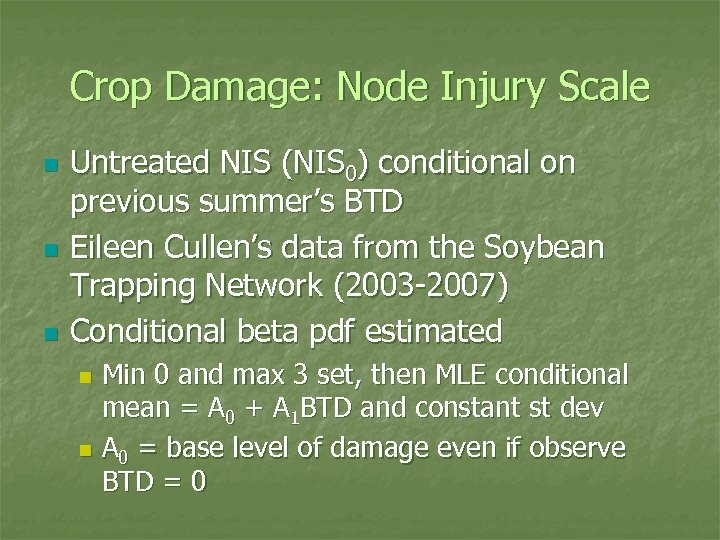Crop Damage: Node Injury Scale n n n Untreated NIS (NIS 0) conditional on previous summer’s BTD Eileen Cullen’s data from the Soybean Trapping Network (2003 -2007) Conditional beta pdf estimated Min 0 and max 3 set, then MLE conditional mean = A 0 + A 1 BTD and constant st dev n A 0 = base level of damage even if observe BTD = 0 n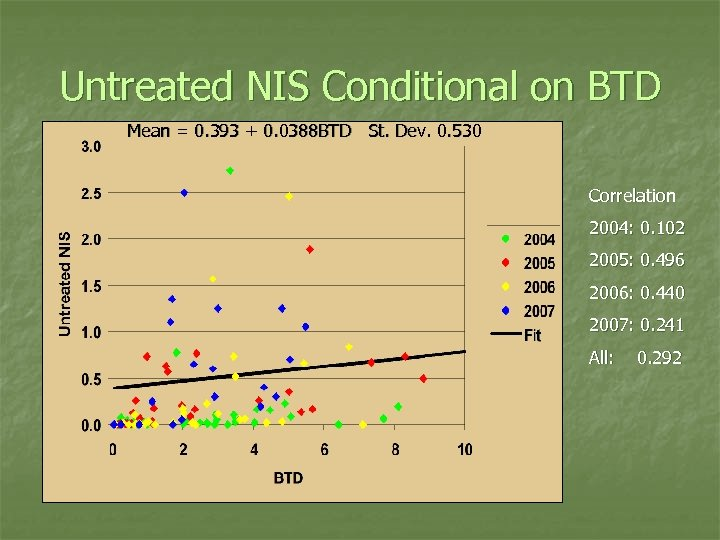Untreated NIS Conditional on BTD Mean = 0. 393 + 0. 0388 BTD St. Dev. 0. 530 Correlation 2004: 0. 102 2005: 0. 496 2006: 0. 440 2007: 0. 241 All: 0. 292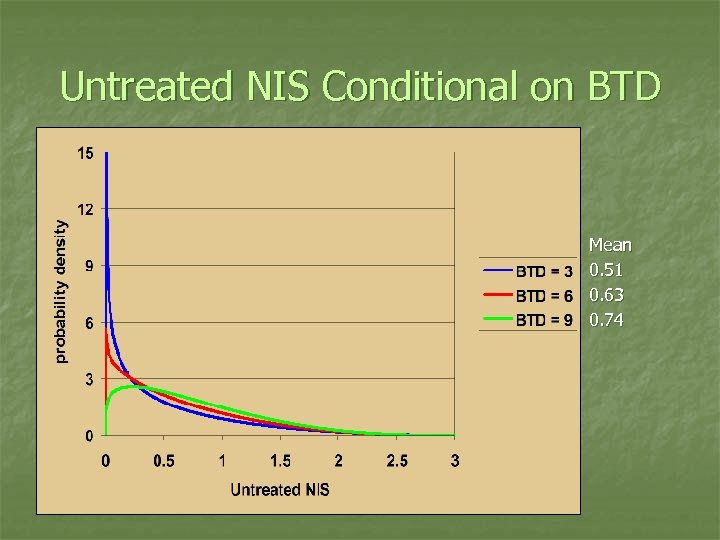Untreated NIS Conditional on BTD Mean 0. 51 0. 63 0. 74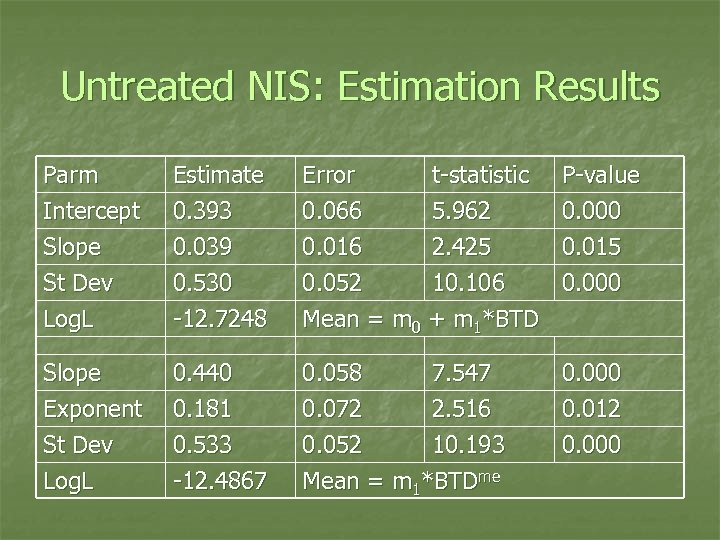Untreated NIS: Estimation Results Parm Intercept Slope St Dev Estimate 0. 393 0. 039 0. 530 Error 0. 066 0. 016 0. 052 t-statistic 5. 962 2. 425 10. 106 Log. L -12. 7248 Mean = m 0 + m 1*BTD Slope Exponent St Dev Log. L 0. 440 0. 181 0. 533 -12. 4867 0. 058 7. 547 0. 072 2. 516 0. 052 10. 193 Mean = m 1*BTDme P-value 0. 000 0. 015 0. 000 0. 012 0. 000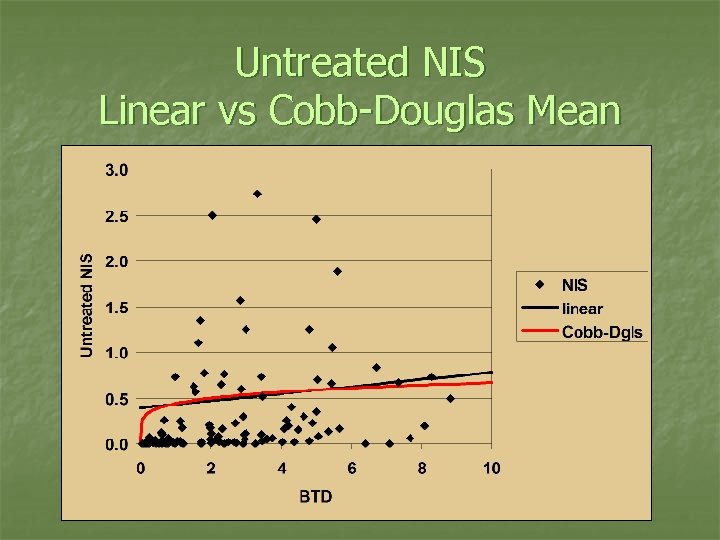Untreated NIS Linear vs Cobb-Douglas Mean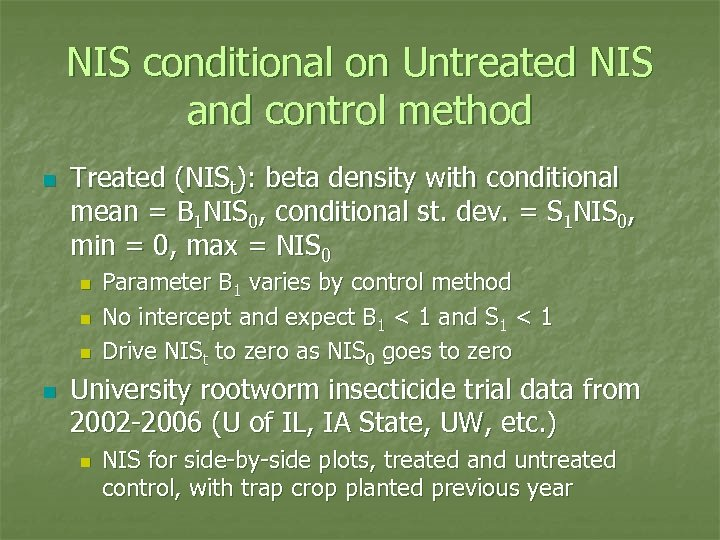NIS conditional on Untreated NIS and control method n Treated (NISt): beta density with conditional mean = B 1 NIS 0, conditional st. dev. = S 1 NIS 0, min = 0, max = NIS 0 n n Parameter B 1 varies by control method No intercept and expect B 1 < 1 and S 1 < 1 Drive NISt to zero as NIS 0 goes to zero University rootworm insecticide trial data from 2002 -2006 (U of IL, IA State, UW, etc. ) n NIS for side-by-side plots, treated and untreated control, with trap crop planted previous year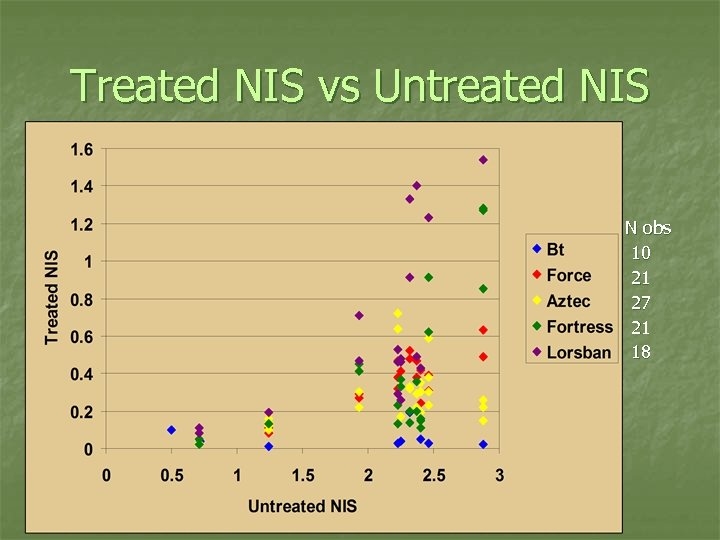Treated NIS vs Untreated NIS N obs 10 21 27 21 18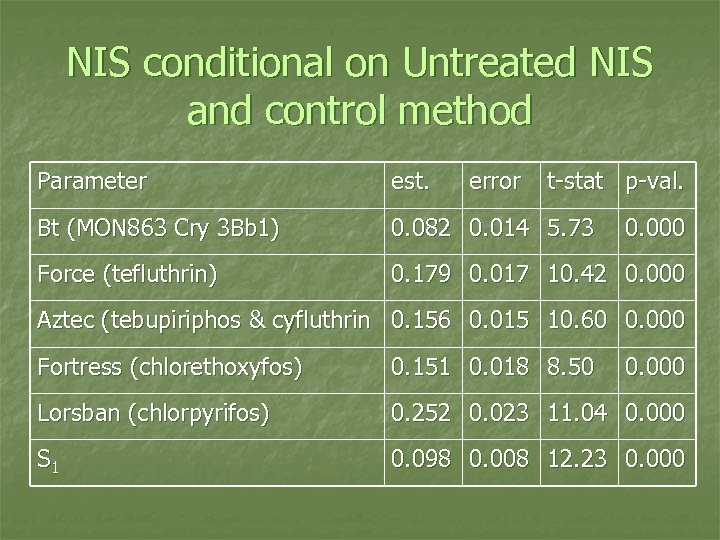NIS conditional on Untreated NIS and control method Parameter est. error t-stat p-val. Bt (MON 863 Cry 3 Bb 1) 0. 082 0. 014 5. 73 Force (tefluthrin) 0. 179 0. 017 10. 42 0. 000 Aztec (tebupiriphos & cyfluthrin 0. 156 0. 015 10. 60 0. 000 Fortress (chlorethoxyfos) 0. 151 0. 018 8. 50 0. 000 Lorsban (chlorpyrifos) 0. 252 0. 023 11. 04 0. 000 S 1 0. 098 0. 008 12. 23 0. 000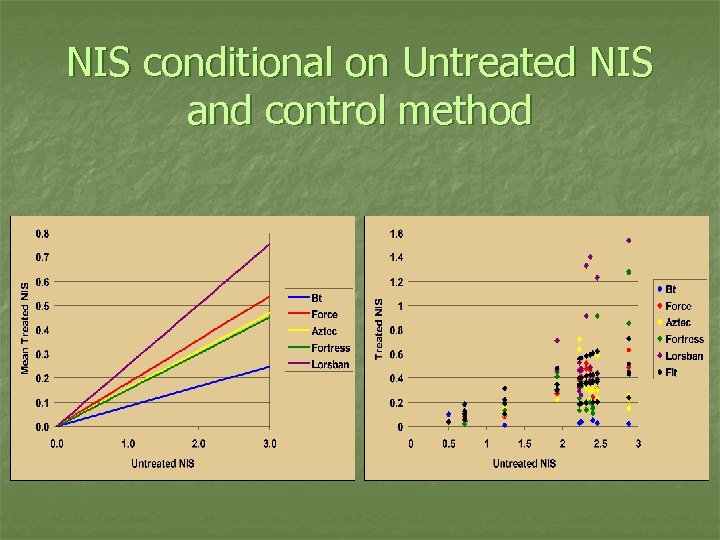NIS conditional on Untreated NIS and control method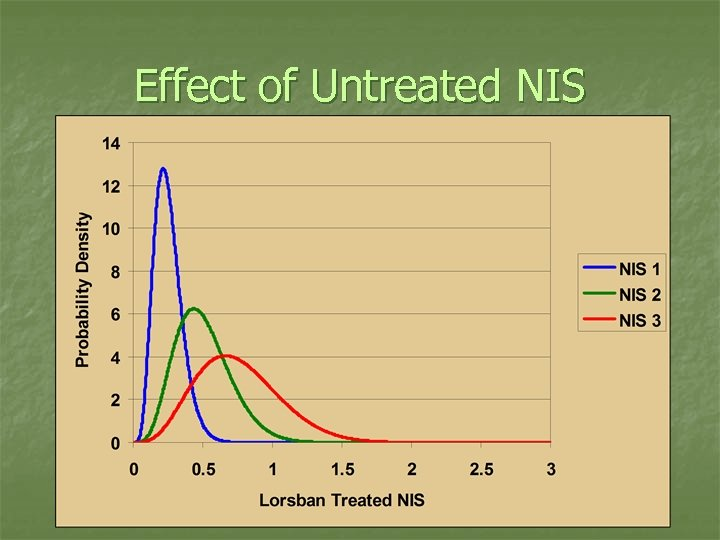Effect of Untreated NIS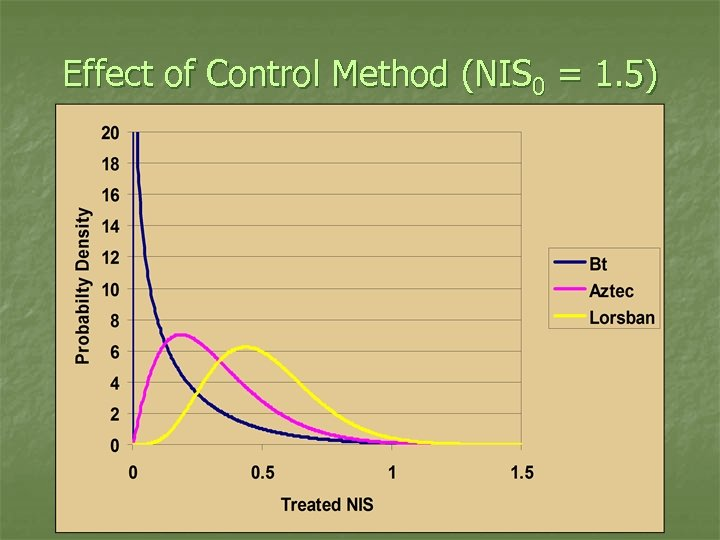Effect of Control Method (NIS 0 = 1. 5)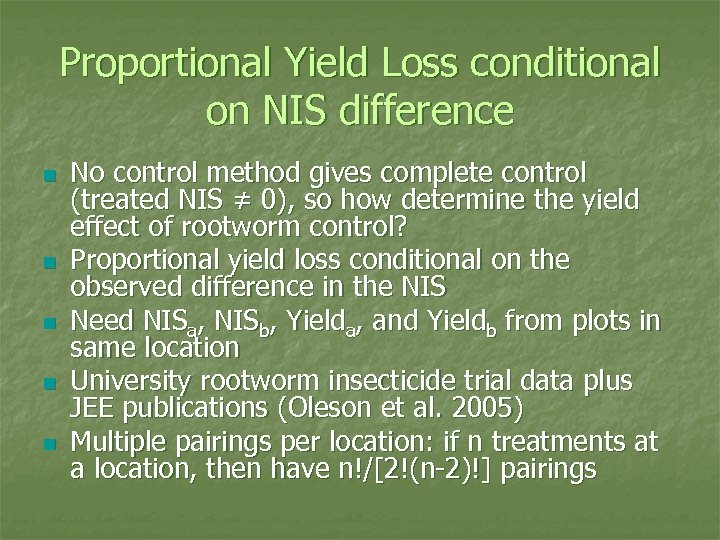Proportional Yield Loss conditional on NIS difference n n n No control method gives complete control (treated NIS ≠ 0), so how determine the yield effect of rootworm control? Proportional yield loss conditional on the observed difference in the NIS Need NISa, NISb, Yielda, and Yieldb from plots in same location University rootworm insecticide trial data plus JEE publications (Oleson et al. 2005) Multiple pairings per location: if n treatments at a location, then have n!/[2!(n-2)!] pairings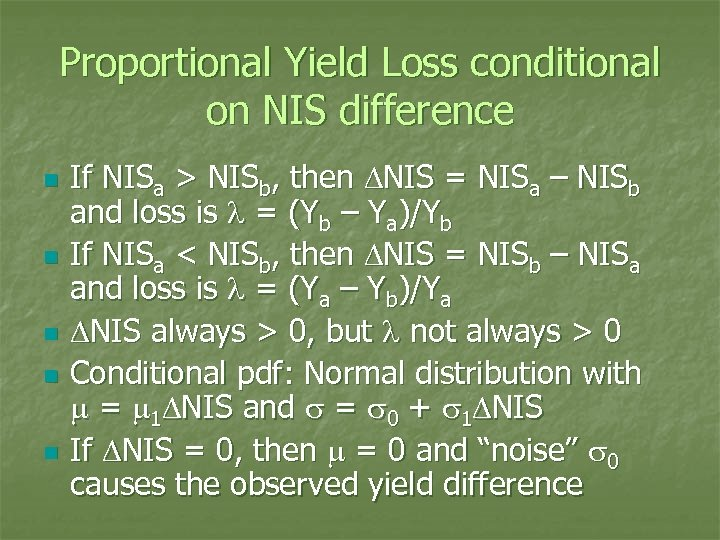Proportional Yield Loss conditional on NIS difference n n n If NISa > NISb, then DNIS = NISa – NISb and loss is l = (Yb – Ya)/Yb If NISa < NISb, then DNIS = NISb – NISa and loss is l = (Ya – Yb)/Ya DNIS always > 0, but l not always > 0 Conditional pdf: Normal distribution with m = m 1 DNIS and s = s 0 + s 1 DNIS If DNIS = 0, then m = 0 and “noise” s 0 causes the observed yield difference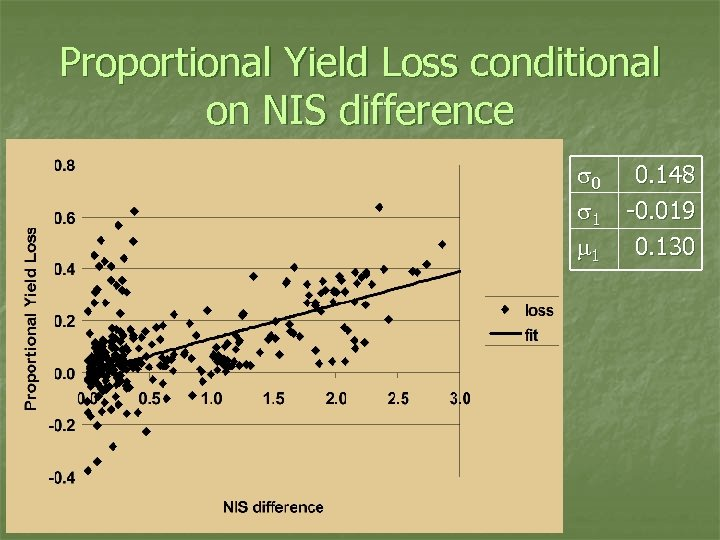Proportional Yield Loss conditional on NIS difference s 0 s 1 m 1 0. 148 -0. 019 0. 130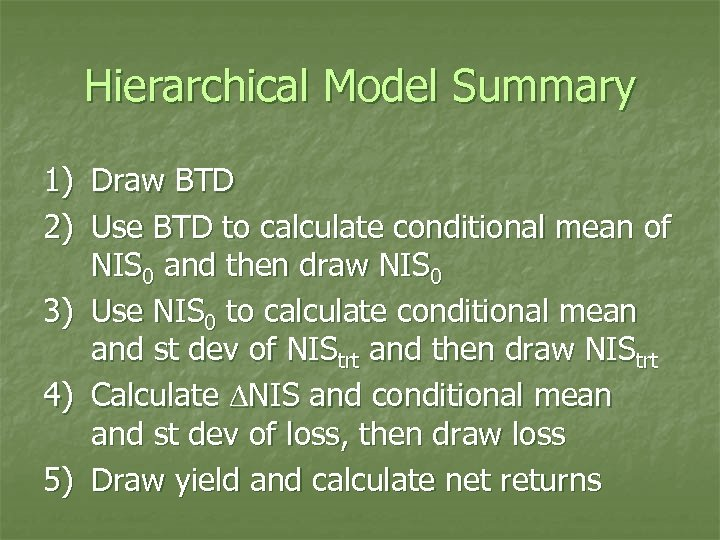Hierarchical Model Summary 1) Draw BTD 2) Use BTD to calculate conditional mean of NIS 0 and then draw NIS 0 3) Use NIS 0 to calculate conditional mean and st dev of NIStrt and then draw NIStrt 4) Calculate DNIS and conditional mean and st dev of loss, then draw loss 5) Draw yield and calculate net returns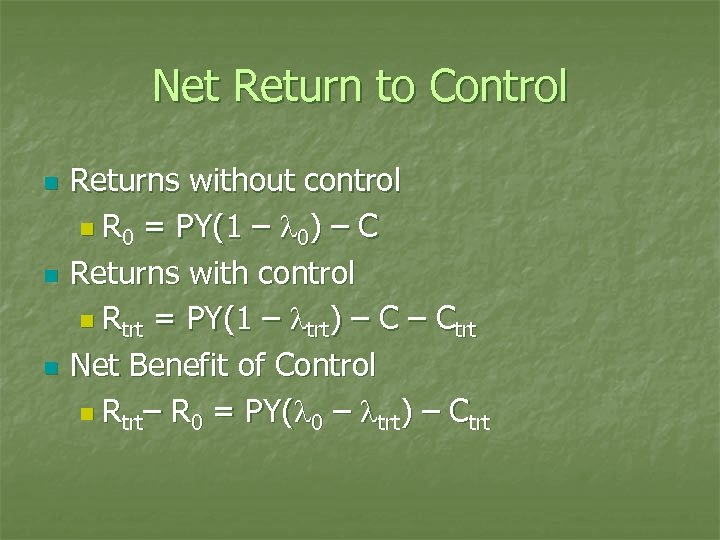Net Return to Control n n n Returns without control n R 0 = PY(1 – l 0) – C Returns with control n Rtrt = PY(1 – ltrt) – Ctrt Net Benefit of Control n Rtrt– R 0 = PY(l 0 – ltrt) – Ctrt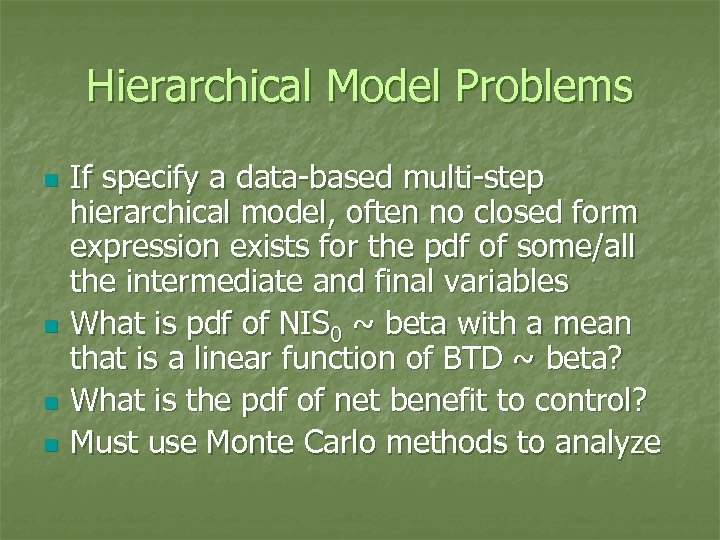Hierarchical Model Problems n n If specify a data-based multi-step hierarchical model, often no closed form expression exists for the pdf of some/all the intermediate and final variables What is pdf of NIS 0 ~ beta with a mean that is a linear function of BTD ~ beta? What is the pdf of net benefit to control? Must use Monte Carlo methods to analyze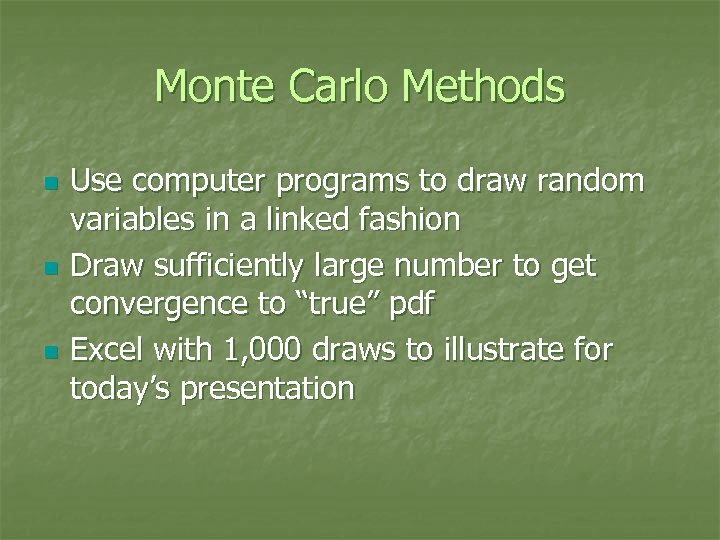Monte Carlo Methods n n n Use computer programs to draw random variables in a linked fashion Draw sufficiently large number to get convergence to “true” pdf Excel with 1, 000 draws to illustrate for today’s presentation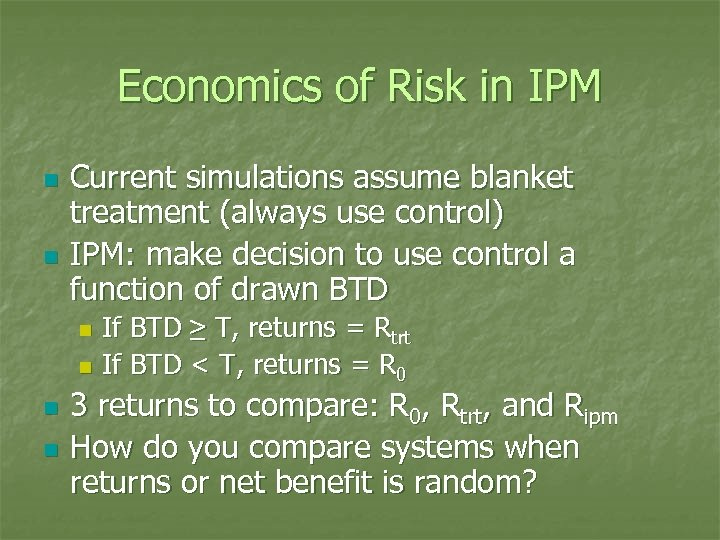Economics of Risk in IPM n n Current simulations assume blanket treatment (always use control) IPM: make decision to use control a function of drawn BTD If BTD ≥ T, returns = Rtrt n If BTD < T, returns = R 0 n n n 3 returns to compare: R 0, Rtrt, and Ripm How do you compare systems when returns or net benefit is random?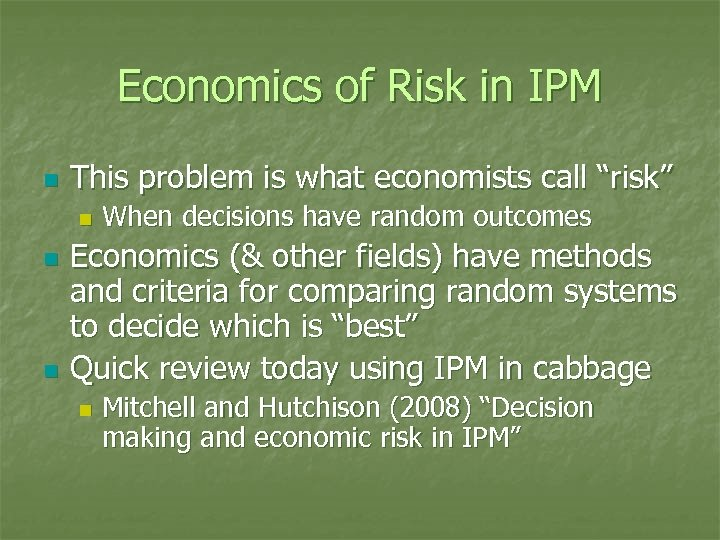Economics of Risk in IPM n This problem is what economists call “risk” n n n When decisions have random outcomes Economics (& other fields) have methods and criteria for comparing random systems to decide which is “best” Quick review today using IPM in cabbage n Mitchell and Hutchison (2008) “Decision making and economic risk in IPM”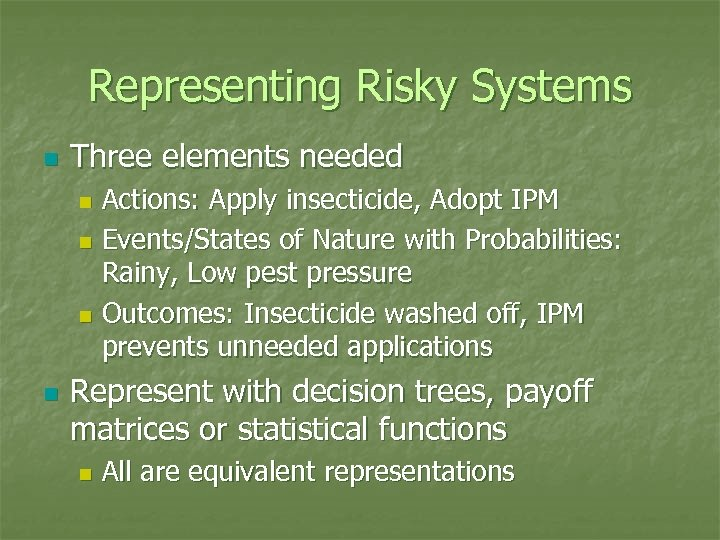Representing Risky Systems n Three elements needed Actions: Apply insecticide, Adopt IPM n Events/States of Nature with Probabilities: Rainy, Low pest pressure n Outcomes: Insecticide washed off, IPM prevents unneeded applications n n Represent with decision trees, payoff matrices or statistical functions n All are equivalent representationsCabbage IPM Case Study n Cabbage looper (Trichoplusia ni) in cabbage (Brassica oleracea) n n Mitchell and Hutchison (2008) Book Chapter Based on Minnesota cabbage IPM 19982001 case study n Hutchison et al. 2006 ab; Burkness & Hutchison 2008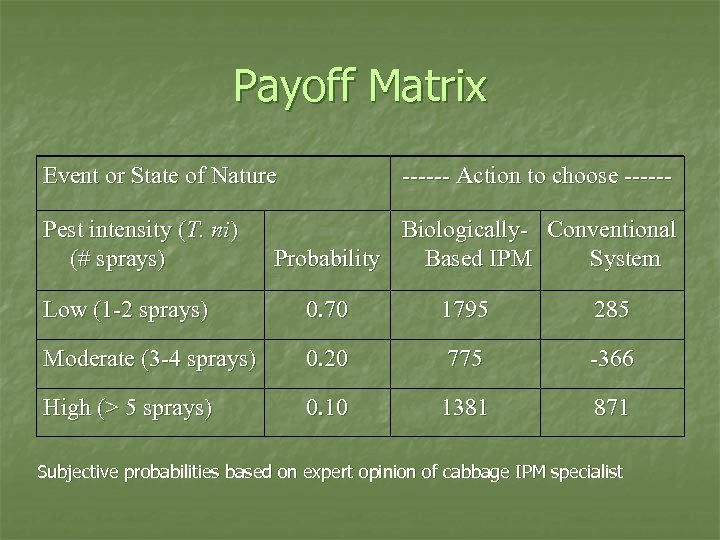Payoff Matrix Event or State of Nature Pest intensity (T. ni) (# sprays) ------ Action to choose ------ Biologically- Conventional Probability Based IPM System Low (1 -2 sprays) 0. 70 1795 285 Moderate (3 -4 sprays) 0. 20 775 -366 High (> 5 sprays) 0. 10 1381 871 Subjective probabilities based on expert opinion of cabbage IPM specialist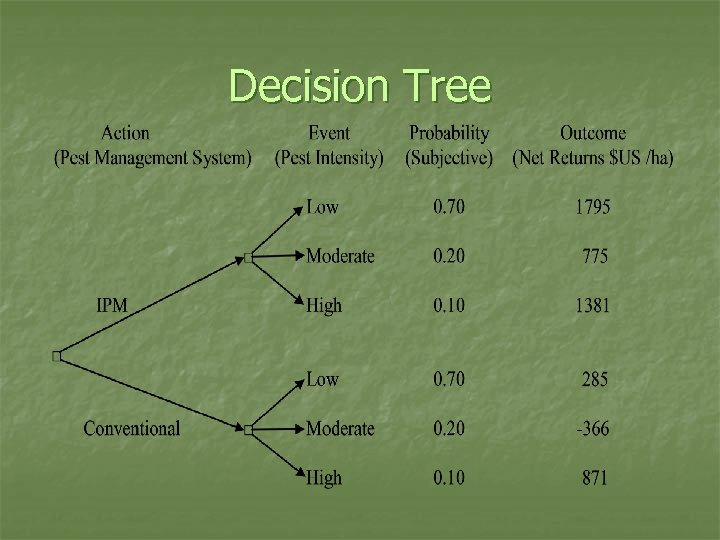Decision Tree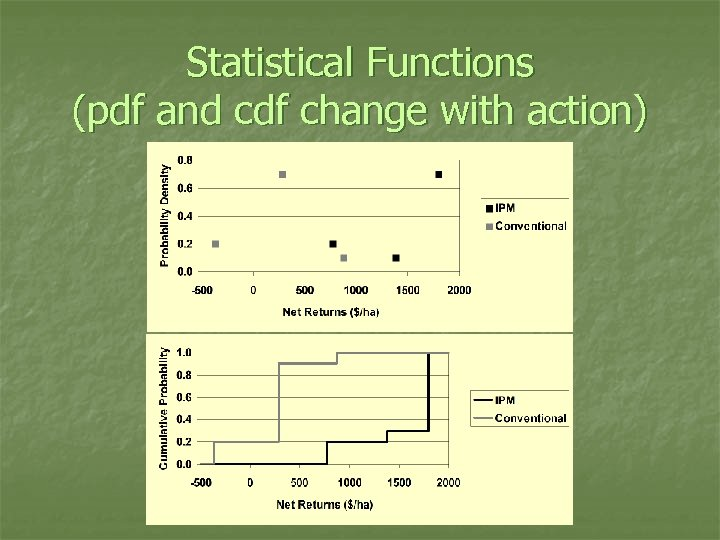Statistical Functions (pdf and cdf change with action)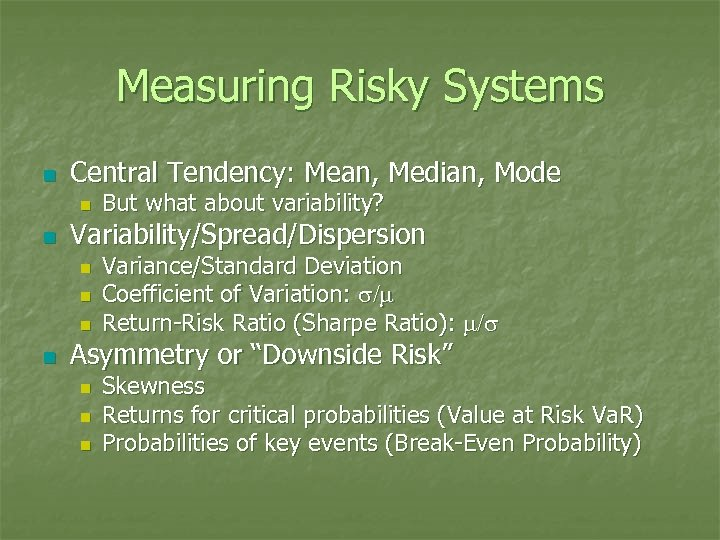Measuring Risky Systems n Central Tendency: Mean, Median, Mode n n Variability/Spread/Dispersion n n But what about variability? Variance/Standard Deviation Coefficient of Variation: s/m Return-Risk Ratio (Sharpe Ratio): m/s Asymmetry or “Downside Risk” n n n Skewness Returns for critical probabilities (Value at Risk Va. R) Probabilities of key events (Break-Even Probability)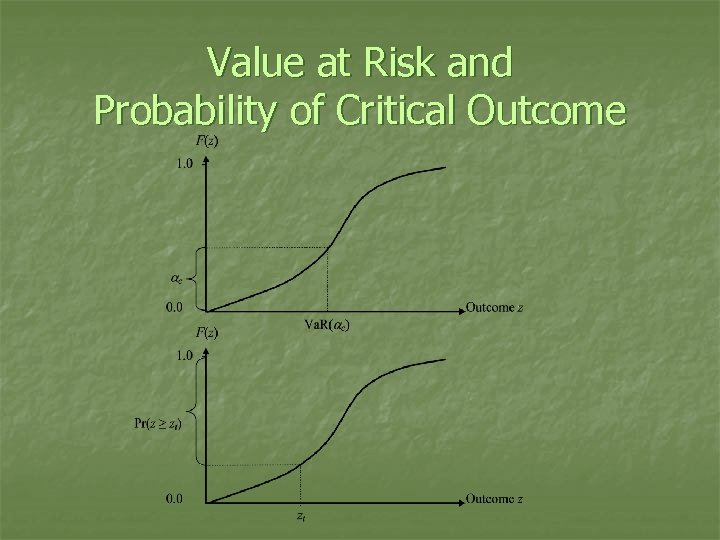Value at Risk and Probability of Critical Outcome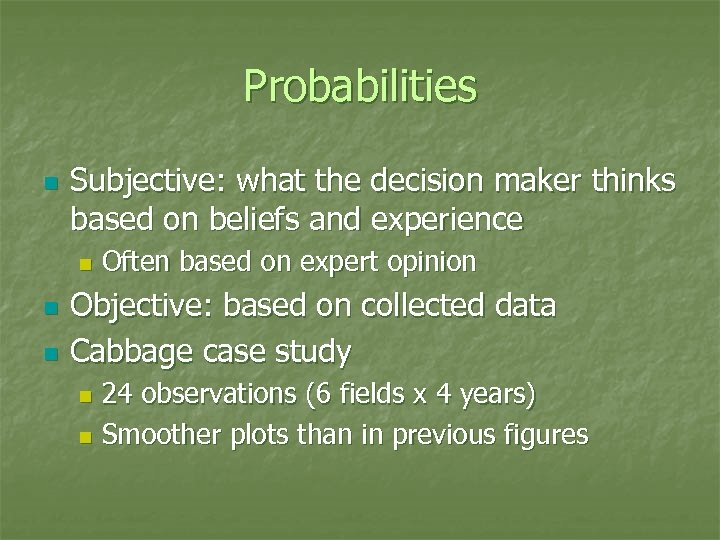Probabilities n Subjective: what the decision maker thinks based on beliefs and experience n n n Often based on expert opinion Objective: based on collected data Cabbage case study 24 observations (6 fields x 4 years) n Smoother plots than in previous figures n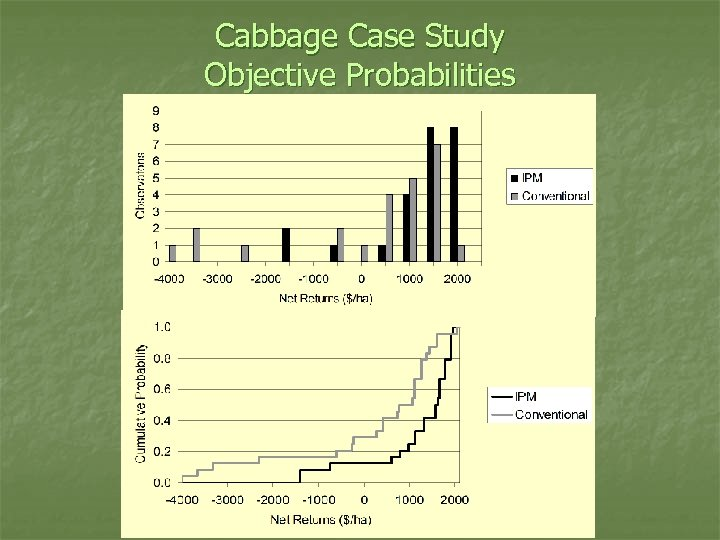Cabbage Case Study Objective Probabilities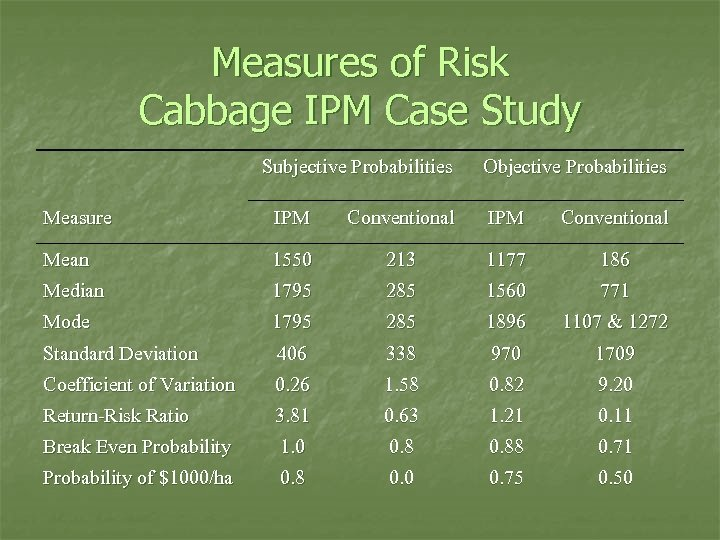Measures of Risk Cabbage IPM Case Study Subjective Probabilities Objective Probabilities Measure IPM Conventional Mean 1550 213 1177 186 Median 1795 285 1560 771 Mode 1795 285 1896 1107 & 1272 Standard Deviation 406 338 970 1709 Coefficient of Variation 0. 26 1. 58 0. 82 9. 20 Return-Risk Ratio 3. 81 0. 63 1. 21 0. 11 Break Even Probability 1. 0 0. 88 0. 71 Probability of \$1000/ha 0. 8 0. 0 0. 75 0. 50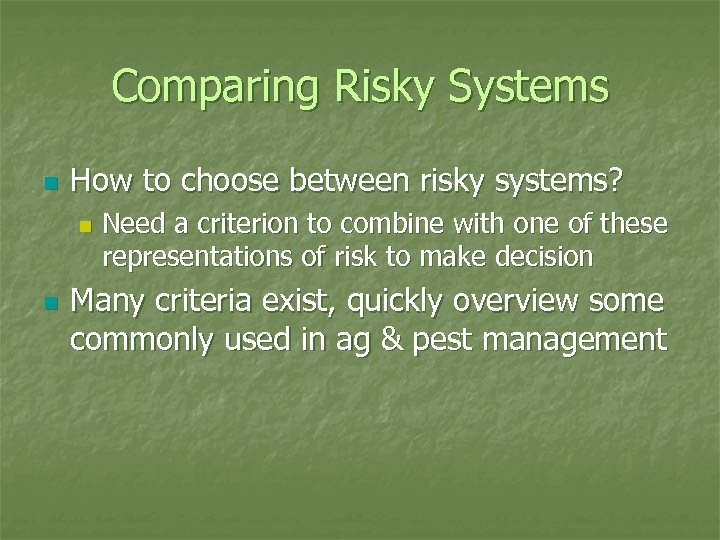Comparing Risky Systems n How to choose between risky systems? n n Need a criterion to combine with one of these representations of risk to make decision Many criteria exist, quickly overview some commonly used in ag & pest management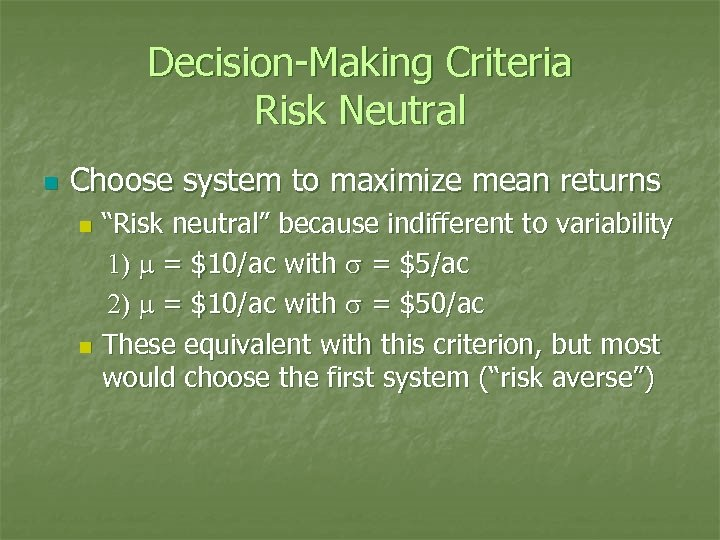Decision-Making Criteria Risk Neutral n Choose system to maximize mean returns “Risk neutral” because indifferent to variability 1) m = \$10/ac with s = \$5/ac 2) m = \$10/ac with s = \$50/ac n These equivalent with this criterion, but most would choose the first system (“risk averse”) n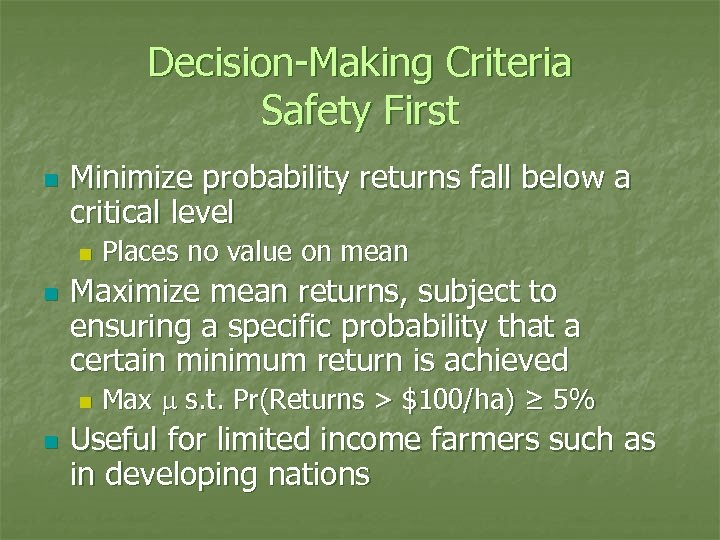Decision-Making Criteria Safety First n Minimize probability returns fall below a critical level n n Maximize mean returns, subject to ensuring a specific probability that a certain minimum return is achieved n n Places no value on mean Max m s. t. Pr(Returns > \$100/ha) ≥ 5% Useful for limited income farmers such as in developing nations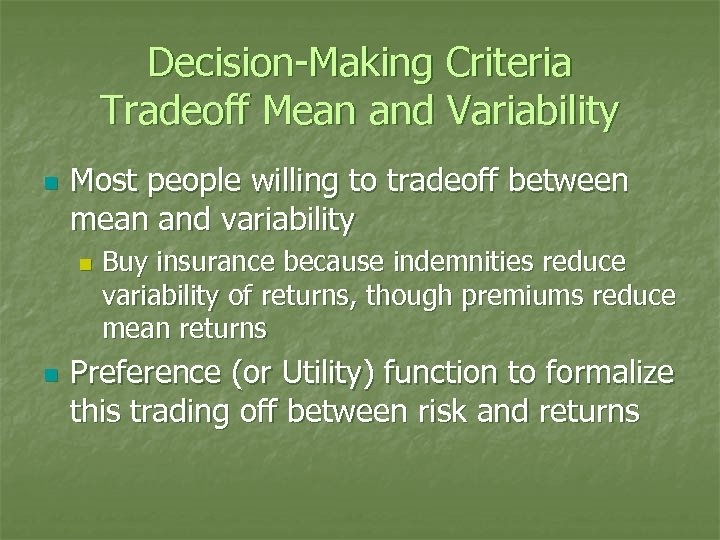Decision-Making Criteria Tradeoff Mean and Variability n Most people willing to tradeoff between mean and variability n n Buy insurance because indemnities reduce variability of returns, though premiums reduce mean returns Preference (or Utility) function to formalize this trading off between risk and returns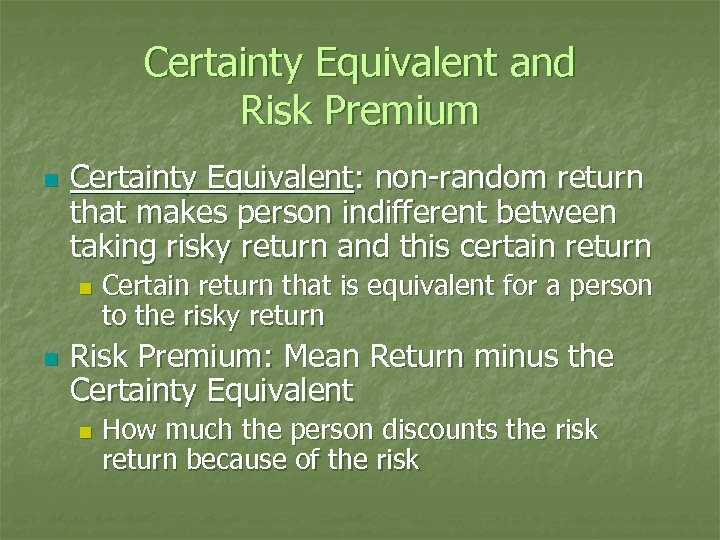Certainty Equivalent and Risk Premium n Certainty Equivalent: non-random return that makes person indifferent between taking risky return and this certain return n n Certain return that is equivalent for a person to the risky return Risk Premium: Mean Return minus the Certainty Equivalent n How much the person discounts the risk return because of the risk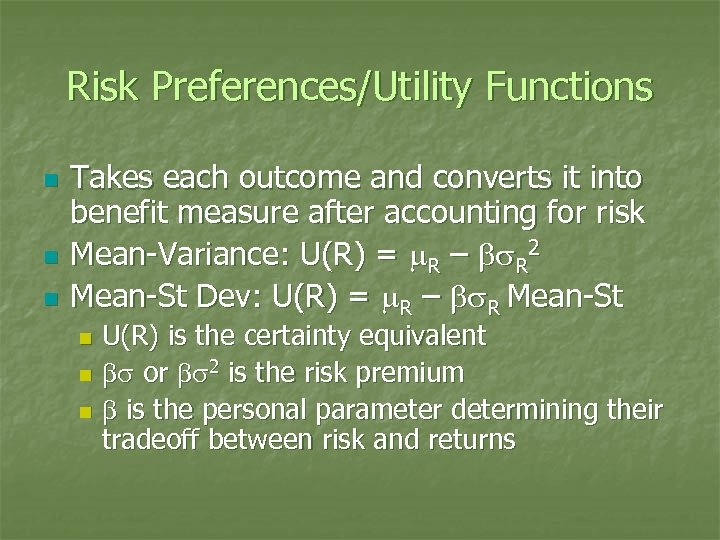Risk Preferences/Utility Functions n n n Takes each outcome and converts it into benefit measure after accounting for risk Mean-Variance: U(R) = m. R – bs. R 2 Mean-St Dev: U(R) = m. R – bs. R Mean-St U(R) is the certainty equivalent n bs or bs 2 is the risk premium n b is the personal parameter determining their tradeoff between risk and returns n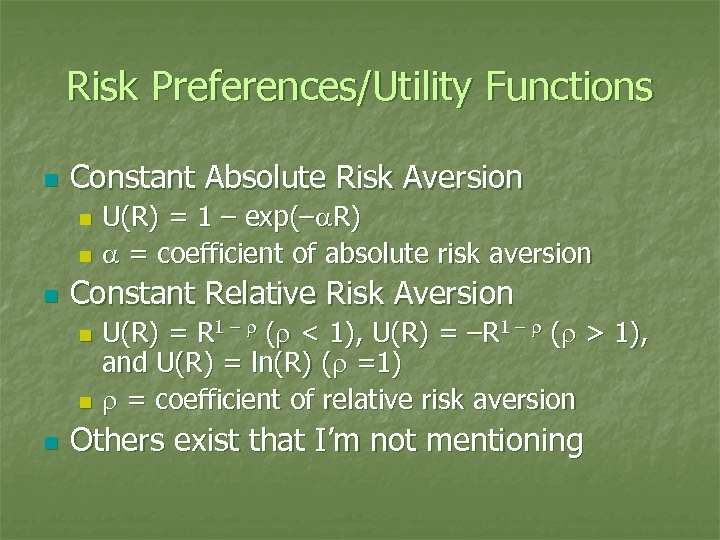Risk Preferences/Utility Functions n Constant Absolute Risk Aversion U(R) = 1 – exp(–a. R) n a = coefficient of absolute risk aversion n n Constant Relative Risk Aversion U(R) = R 1 – r (r < 1), U(R) = –R 1 – r (r > 1), and U(R) = ln(R) (r =1) n r = coefficient of relative risk aversion n n Others exist that I’m not mentioning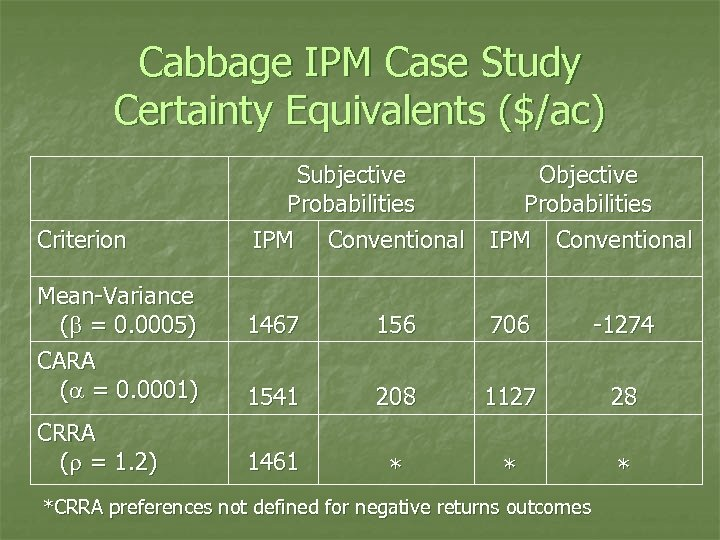Cabbage IPM Case Study Certainty Equivalents (\$/ac) Criterion Mean-Variance (b = 0. 0005) CARA (a = 0. 0001) CRRA (r = 1. 2) Subjective Probabilities IPM Conventional Objective Probabilities IPM Conventional 1467 156 706 -1274 1541 208 1127 28 1461 * *CRRA preferences not defined for negative returns outcomes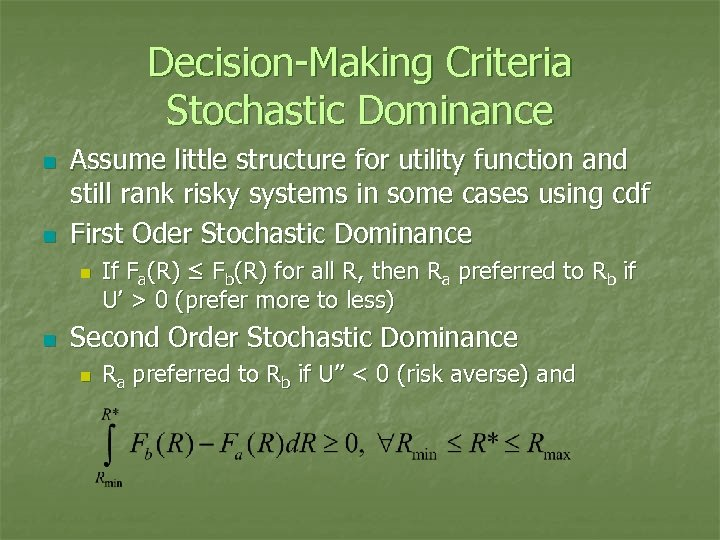Decision-Making Criteria Stochastic Dominance n n Assume little structure for utility function and still rank risky systems in some cases using cdf First Oder Stochastic Dominance n n If Fa(R) ≤ Fb(R) for all R, then Ra preferred to Rb if U’ > 0 (prefer more to less) Second Order Stochastic Dominance n Ra preferred to Rb if U’’ < 0 (risk averse) and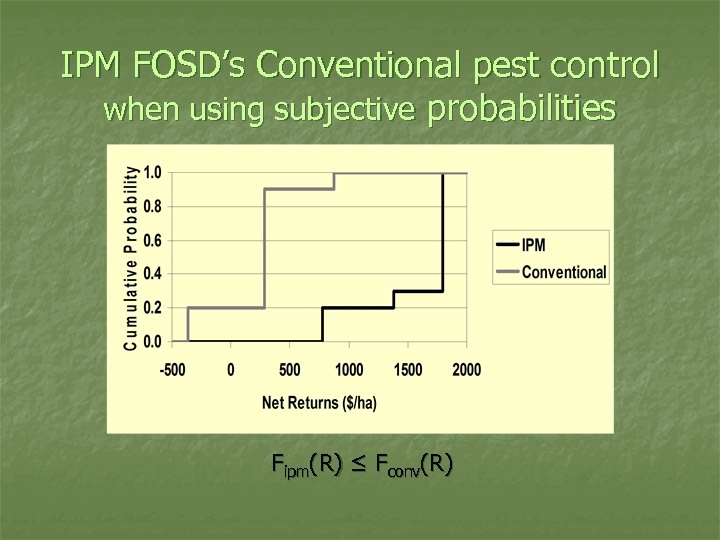IPM FOSD’s Conventional pest control when using subjective probabilities Fipm(R) ≤ Fconv(R)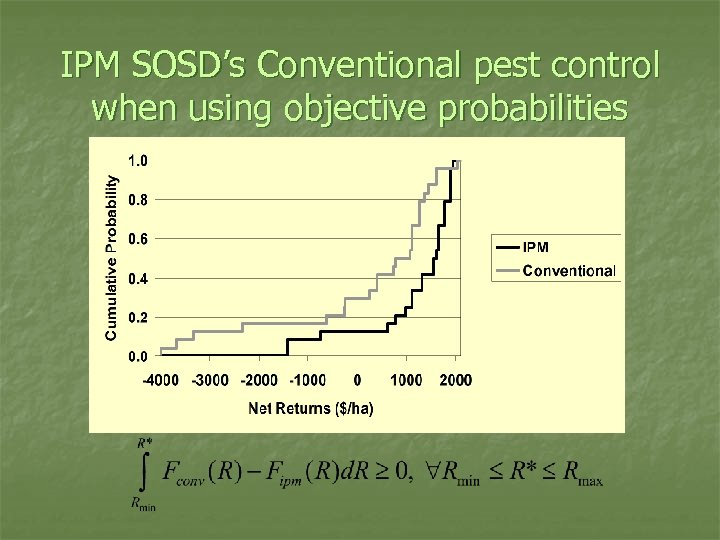IPM SOSD’s Conventional pest control when using objective probabilities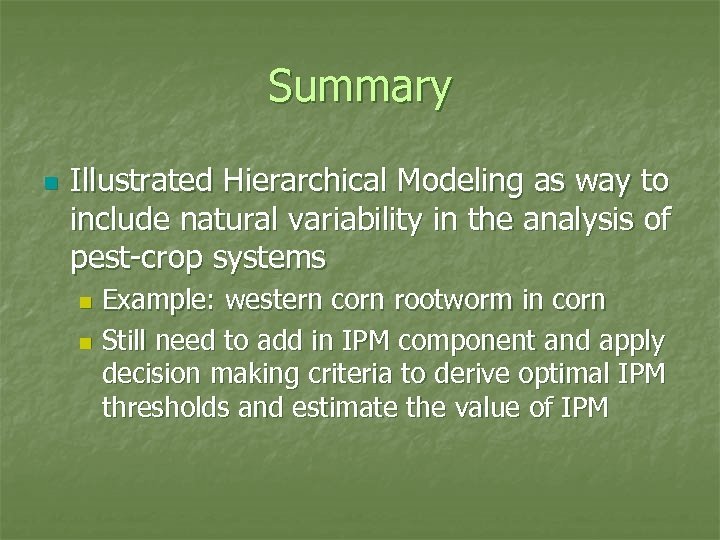Summary n Illustrated Hierarchical Modeling as way to include natural variability in the analysis of pest-crop systems Example: western corn rootworm in corn n Still need to add in IPM component and apply decision making criteria to derive optimal IPM thresholds and estimate the value of IPM n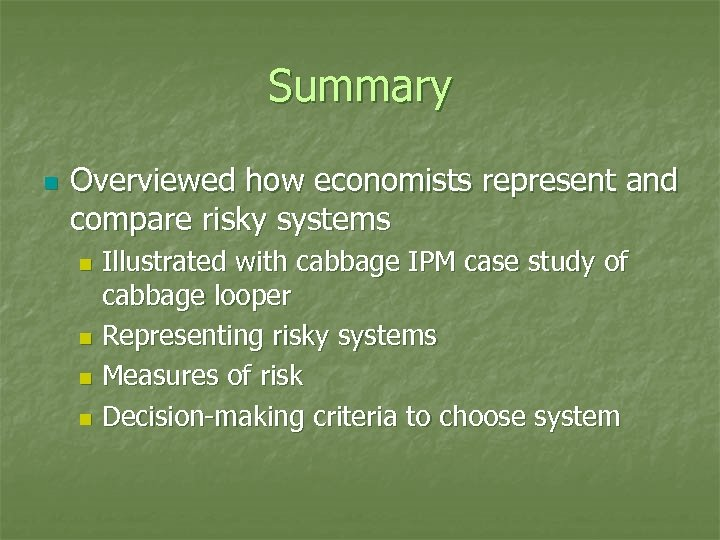Summary n Overviewed how economists represent and compare risky systems Illustrated with cabbage IPM case study of cabbage looper n Representing risky systems n Measures of risk n Decision-making criteria to choose system n